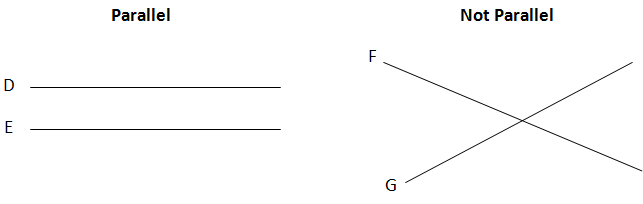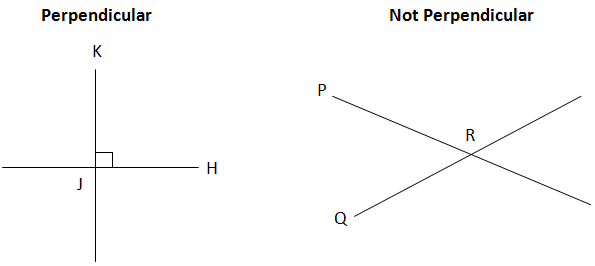# GMAT Quant: Geometry – LinesGMAT Quant analyses your mathematical skills based on many topics of Geometry like Triangle, Special Right Triangles, Area, Perimeter, and Volume of various geometrical shapes etc.

What is a Line?

A line is a series of joined dots that extend to infinity.

So, if a line has no end points, how do we operate over it?

We just take a segment of the line of some particular length that fulfills our requirements. These segments of lines are known as line segments.Some special types of lines:

## Parallel Lines

Two lines lying in the same plane yet never intersecting one another is said to be parallel lines. From the concept of co-ordinate geometry, two parallel lines will have the same slope but different intercepts related to the plane they are lying in. Whereas, non-parallel lines intersect one another at some point and have different slopes.## Perpendicular Lines

Perpendicular lines are intersecting lines that intersect at an exact angle of 90 Degrees.These were some of the basic information about lines.

Let’s solve a question now:If AB & ED are parallel to one another, then,

What is the value of angle C?

Solution:Step1. As AB and ED are parallel,

So, angle EAO = angle AED = 65 degree

Step2. Angle OAE + angle EAD + angle DAB = 180 degree

So, angle DAB= 40 degrees

Step3. Angle CAB + angle ABC + angle BCA = 180 degree

So, angle C = 45 degree.

We’ll be glad to help you in your GMAT preparation journey. You can ask for any assistance related to GMAT and MBA from us by calling us at +918884544444. You can write to us at gmat@byjus.com.